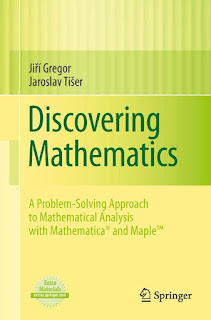ANALYSICS MATHEMATICS

# Discovering Mathematics A Problem Solving Approach to Mathematical Analysis with MATHEMATICA® and Maple™ PDF Free DownloadThe book contains chapters of structured approach to problem solving in mathematical analysis on an intermediate level. It follows the ideas of G.Polya and others, distinguishing between exercises and problem solving in mathematics. Interrelated concepts are connected by hyperlinks, pointing toward easier or more difficult problems so as to show paths of mathematical reasoning. Basic definitions and theorems can also be found by hyperlinks from relevant places. Problems are open to alternative formulations, generalizations, simplifications, and verification of hypotheses by the reader; this is shown to be helpful in solving problems. The book presents how advanced mathematical software can aid all stages of mathematical reasoning while the mathematical content remains in foreground. The authors show how software can contribute to deeper understanding and to enlarging the scope of teaching for students and teachers of mathematics.